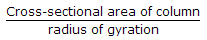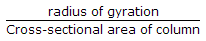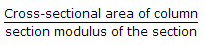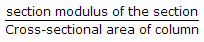Civil Engineering - Steel Structure Design - Discussion

Discussion :: Steel Structure Design - Section 2 (Q.No.43)

43.

For eccentrically loaded columns, the bending factor is

 [A].[B].[C].[D].Explanation:

No answer description available for this question.

 Raj said: (Dec 8, 2014) We know M/I = F/y. So M = F*Z, Z section modulus. Therefore we can write M = P*(Z/A). May be answer is D.

 Michael said: (Oct 4, 2016) What is the right answer anyone know?

 Garry said: (Jan 19, 2018) I totally agree @ Raj the correct answer should be option d.

 Uttudon said: (Oct 3, 2018) Z/A is the correct answer. So, Option D is correct.

 Pooja said: (Dec 22, 2019) M/I = f/y f = M X y/I = M /Z Load = M * A/Z so answer is C.

 Ashish Sharma said: (Sep 15, 2020) D is the correct answer.